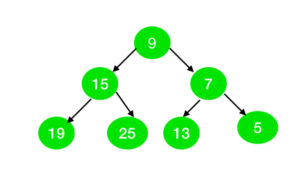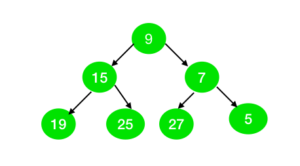# Check if all nodes of the Binary Tree can be represented as sum of two primes

Given a binary tree of N nodes with odd value. The task is to check whether all the nodes of the tree can be represented as the sum of the two prime numbers or not.
Examples:

Input:Output: Yes
Explanation:
All the nodes in the tree can be represented as the sum of two prime numbers as:
9 = 2 + 7
15 = 2 +13
7 = 2 + 5
19 = 2 + 17
25 = 2 + 23
13 = 11 + 2
5 = 2 + 3

Input:Output: No
Explanation:
The node with value 27 cannot be represented as the sum of two prime numbers.

Approach:

1. The idea is to use Goldbach’s Weak Conjecture which states that every odd number greater than 5 can be expressed as the sum of three primes.
2. To represent odd number(say N) as a sum of two prime numbers, fix one prime number as 2 and if (N – 2) is also prime, then N can be represented as a sum of two prime numbers.
3. Check the above condition for all the nodes in a tree. If any node doesn’t follow the above condition then print “No” else print “Yes”.

## C++

 `// C++ program for the above approach ` `#include ` `using` `namespace` `std; ` ` `  `// Function to create array to mark ` `// whether element are prime or not ` `void` `spf_array(``int` `arr[], ``int` `N) ` `{ ` `    ``int` `i = 0; ` ` `  `    ``// Initially we set same value in ` `    ``// array as a index of array. ` `    ``for` `(i = 1; i <= N; i++) { ` `        ``arr[i] = i; ` `    ``} ` ` `  `    ``// Mark all even elements as 2 ` `    ``for` `(i = 2; i <= N; i = i + 2) { ` `        ``arr[i] = 2; ` `    ``} ` ` `  `    ``// Mark all the multiple of prime ` `    ``// numbers as a non-prime ` `    ``for` `(i = 3; i * i <= N; i++) { ` `        ``if` `(arr[i] == i) { ` ` `  `            ``int` `j = 0; ` ` `  `            ``for` `(j = i * i; j <= N; ` `                 ``j = j + i) { ` ` `  `                ``if` `(arr[j] == j) { ` `                    ``arr[j] = i; ` `                ``} ` `            ``} ` `        ``} ` `    ``} ` `} ` ` `  `// Tree Node ` `struct` `node { ` `    ``int` `val; ` `    ``node* left; ` `    ``node* right; ` `}; ` ` `  `// Funtion to create node of tree ` `node* newnode(``int` `i) ` `{ ` `    ``node* temp = NULL; ` `    ``temp = ``new` `node(); ` `    ``temp->val = i; ` `    ``temp->left = NULL; ` `    ``temp->right = NULL; ` `    ``return` `temp; ` `} ` ` `  `// Function to check whether the ` `// tree is prime or not ` `int` `prime_tree(node* root, ``int` `arr[]) ` `{ ` `    ``int` `a = -1; ` `    ``if` `(root != NULL) { ` ` `  `        ``// If element is not the sum of ` `        ``// two prime then return 0 ` `        ``if` `(root->val <= 3 ` `            ``|| arr[root->val - 2] ` `                   ``!= root->val - 2) { ` ` `  `            ``return` `0; ` `        ``} ` `    ``} ` ` `  `    ``if` `(root->left != NULL) { ` `        ``a = prime_tree(root->left, arr); ` ` `  `        ``// If a is 0 then we don't need ` `        ``// to check further ` `        ``if` `(a == 0) { ` `            ``return` `0; ` `        ``} ` `    ``} ` ` `  `    ``if` `(root->right != NULL) { ` ` `  `        ``a = prime_tree(root->right, arr); ` ` `  `        ``// If a is 0 then we don't need ` `        ``// to check further ` `        ``if` `(a == 0) { ` `            ``return` `0; ` `        ``} ` `    ``} ` ` `  `    ``return` `1; ` `} ` ` `  `// Driver Code ` `int` `main() ` `{ ` ` `  `    ``// Given Tree ` `    ``node* root = newnode(9); ` `    ``root->right = newnode(7); ` `    ``root->right->right = newnode(5); ` `    ``root->right->left = newnode(13); ` `    ``root->left = newnode(15); ` `    ``root->left->left = newnode(19); ` `    ``root->left->right = newnode(25); ` ` `  `    ``// Number of nodes in the tree ` `    ``int` `n = 50; ` ` `  `    ``// Declare spf[] to store ` `    ``// prime numbers ` `    ``int` `brr[n + 1]; ` `    ``int` `i = 0; ` ` `  `    ``// Find prime numbers in spf[] ` `    ``spf_array(brr, n + 1); ` ` `  `    ``// Function Call ` `    ``if` `(prime_tree(root, brr)) { ` `        ``cout << ``"Yes"` `<< endl; ` `    ``} ` `    ``else` `{ ` `        ``cout << ``"No"` `<< endl; ` `    ``} ` `} `

## Java

 `// Java program for the above approach ` `import` `java.util.*; ` ` `  `class` `GFG{ ` ` `  `// Function to create array to mark ` `// whether element are prime or not ` `static` `void` `spf_array(``int` `arr[], ``int` `N) ` `{ ` `    ``int` `i = ``0``; ` ` `  `    ``// Initially we set same value in ` `    ``// array as a index of array. ` `    ``for``(i = ``1``; i <= N; i++) ` `    ``{ ` `        ``arr[i] = i; ` `    ``} ` ` `  `    ``// Mark all even elements as 2 ` `    ``for``(i = ``2``; i <= N; i = i + ``2``) ` `    ``{ ` `        ``arr[i] = ``2``; ` `    ``} ` ` `  `    ``// Mark all the multiple of prime ` `    ``// numbers as a non-prime ` `    ``for``(i = ``3``; i * i <= N; i++)  ` `    ``{ ` `        ``if` `(arr[i] == i)  ` `        ``{ ` `            ``int` `j = ``0``; ` `            ``for``(j = i * i; j <= N;  ` `                ``j = j + i) ` `            ``{ ` `                ``if` `(arr[j] == j)  ` `                ``{ ` `                    ``arr[j] = i; ` `                ``} ` `            ``} ` `        ``} ` `    ``} ` `} ` ` `  `// Tree Node ` `static` `class` `node ` `{ ` `    ``int` `val; ` `    ``node left; ` `    ``node right; ` `}; ` ` `  `// Funtion to create node of tree ` `static` `node newnode(``int` `i) ` `{ ` `    ``node temp = ``null``; ` `    ``temp = ``new` `node(); ` `    ``temp.val = i; ` `    ``temp.left = ``null``; ` `    ``temp.right = ``null``; ` `    ``return` `temp; ` `} ` ` `  `// Function to check whether  ` `// the tree is prime or not ` `static` `int` `prime_tree(node root, ``int` `arr[]) ` `{ ` `    ``int` `a = -``1``; ` `    ``if` `(root != ``null``)  ` `    ``{ ` `         `  `        ``// If element is not the sum  ` `        ``// of two prime then return 0 ` `        ``if` `(root.val <= ``3` `||  ` `         ``arr[root.val - ``2``] !=  ` `             ``root.val - ``2``)  ` `        ``{ ` `            ``return` `0``; ` `        ``} ` `    ``} ` `     `  `    ``if` `(root.left != ``null``) ` `    ``{ ` `        ``a = prime_tree(root.left, arr); ` ` `  `        ``// If a is 0 then we don't  ` `        ``// need to check further ` `        ``if` `(a == ``0``) ` `        ``{ ` `            ``return` `0``; ` `        ``} ` `    ``} ` ` `  `    ``if` `(root.right != ``null``) ` `    ``{ ` `        ``a = prime_tree(root.right, arr); ` ` `  `        ``// If a is 0 then we don't  ` `        ``// need to check further ` `        ``if` `(a == ``0``)  ` `        ``{ ` `            ``return` `0``; ` `        ``} ` `    ``} ` `    ``return` `1``; ` `} ` ` `  `// Driver Code ` `public` `static` `void` `main(String[] args) ` `{ ` `     `  `    ``// Given Tree ` `    ``node root = newnode(``9``); ` `    ``root.right = newnode(``7``); ` `    ``root.right.right = newnode(``5``); ` `    ``root.right.left = newnode(``13``); ` `    ``root.left = newnode(``15``); ` `    ``root.left.left = newnode(``19``); ` `    ``root.left.right = newnode(``25``); ` ` `  `    ``// Number of nodes in the tree ` `    ``int` `n = ``50``; ` ` `  `    ``// Declare spf[] to store ` `    ``// prime numbers ` `    ``int` `[]brr = ``new` `int``[n + ``1``]; ` `    ``int` `i = ``0``; ` ` `  `    ``// Find prime numbers in spf[] ` `    ``spf_array(brr, n); ` ` `  `    ``// Function Call ` `    ``if` `(prime_tree(root, brr) == ``1``)  ` `    ``{ ` `        ``System.out.print(``"Yes"` `+ ``"\n"``); ` `    ``} ` `    ``else` `    ``{ ` `        ``System.out.print(``"No"` `+ ``"\n"``); ` `    ``} ` `} ` `} ` ` `  `// This code is contributed by Rohit_ranjan `

## C#

 `// C# program for the above approach ` `using` `System; ` ` `  `class` `GFG{ ` ` `  `// Function to create array to mark ` `// whether element are prime or not ` `static` `void` `spf_array(``int` `[]arr, ``int` `N) ` `{ ` `    ``int` `i = 0; ` ` `  `    ``// Initially we set same value in ` `    ``// array as a index of array. ` `    ``for``(i = 1; i <= N; i++) ` `    ``{ ` `        ``arr[i] = i; ` `    ``} ` ` `  `    ``// Mark all even elements as 2 ` `    ``for``(i = 2; i <= N; i = i + 2) ` `    ``{ ` `        ``arr[i] = 2; ` `    ``} ` ` `  `    ``// Mark all the multiple of prime ` `    ``// numbers as a non-prime ` `    ``for``(i = 3; i * i <= N; i++)  ` `    ``{ ` `        ``if` `(arr[i] == i)  ` `        ``{ ` `            ``int` `j = 0; ` `            ``for``(j = i * i; j <= N;  ` `                ``j = j + i) ` `            ``{ ` `                ``if` `(arr[j] == j)  ` `                ``{ ` `                    ``arr[j] = i; ` `                ``} ` `            ``} ` `        ``} ` `    ``} ` `} ` ` `  `// Tree Node ` `class` `node ` `{ ` `    ``public` `int` `val; ` `    ``public` `node left; ` `    ``public` `node right; ` `}; ` ` `  `// Funtion to create node of tree ` `static` `node newnode(``int` `i) ` `{ ` `    ``node temp = ``null``; ` `    ``temp = ``new` `node(); ` `    ``temp.val = i; ` `    ``temp.left = ``null``; ` `    ``temp.right = ``null``; ` `    ``return` `temp; ` `} ` ` `  `// Function to check whether  ` `// the tree is prime or not ` `static` `int` `prime_tree(node root, ``int` `[]arr) ` `{ ` `    ``int` `a = -1; ` `    ``if` `(root != ``null``)  ` `    ``{ ` `         `  `        ``// If element is not the sum  ` `        ``// of two prime then return 0 ` `        ``if` `(root.val <= 3 ||  ` `        ``arr[root.val - 2] !=  ` `            ``root.val - 2)  ` `        ``{ ` `            ``return` `0; ` `        ``} ` `    ``} ` `     `  `    ``if` `(root.left != ``null``) ` `    ``{ ` `        ``a = prime_tree(root.left, arr); ` ` `  `        ``// If a is 0 then we don't  ` `        ``// need to check further ` `        ``if` `(a == 0) ` `        ``{ ` `            ``return` `0; ` `        ``} ` `    ``} ` ` `  `    ``if` `(root.right != ``null``) ` `    ``{ ` `        ``a = prime_tree(root.right, arr); ` ` `  `        ``// If a is 0 then we don't  ` `        ``// need to check further ` `        ``if` `(a == 0)  ` `        ``{ ` `            ``return` `0; ` `        ``} ` `    ``} ` `    ``return` `1; ` `} ` ` `  `// Driver Code ` `public` `static` `void` `Main(String[] args) ` `{ ` `     `  `    ``// Given Tree ` `    ``node root = newnode(9); ` `    ``root.right = newnode(7); ` `    ``root.right.right = newnode(5); ` `    ``root.right.left = newnode(13); ` `    ``root.left = newnode(15); ` `    ``root.left.left = newnode(19); ` `    ``root.left.right = newnode(25); ` ` `  `    ``// Number of nodes in the tree ` `    ``int` `n = 50; ` ` `  `    ``// Declare spf[] to store ` `    ``// prime numbers ` `    ``int` `[]brr = ``new` `int``[n + 1]; ` ` `  `    ``// Find prime numbers in spf[] ` `    ``spf_array(brr, n); ` ` `  `    ``// Function Call ` `    ``if` `(prime_tree(root, brr) == 1)  ` `    ``{ ` `        ``Console.Write(``"Yes"` `+ ``"\n"``); ` `    ``} ` `    ``else` `    ``{ ` `        ``Console.Write(``"No"` `+ ``"\n"``); ` `    ``} ` `} ` `} ` ` `  `// This code is contributed by amal kumar choubey `

Output:

```Yes
```

Time complexity : O (N * log(log N))
Auxiliary Space : O (N)

Attention reader! Don’t stop learning now. Get hold of all the important DSA concepts with the DSA Self Paced Course at a student-friendly price and become industry ready.

My Personal Notes arrow_drop_upCheck out this Author's contributed articles.

If you like GeeksforGeeks and would like to contribute, you can also write an article using contribute.geeksforgeeks.org or mail your article to contribute@geeksforgeeks.org. See your article appearing on the GeeksforGeeks main page and help other Geeks.

Please Improve this article if you find anything incorrect by clicking on the "Improve Article" button below.